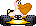#Phil’s Wacky Wheels SiteSkip navigation

## Source code snippets

### Dopefish source code

This piece of source code was posted on the 3D Realms Forums by Andy Edwardson, the programmer of Wacky Wheels. I formatted the code some to make it easier to read than it is on the forum. Notice that the code loads `DOPE.SP` and `BELCH.VOC`, files that you can get by running the Wombat Game Tools. `BELCH.VOC` is a sound file, and you can get a wave file version of it and the other `.VOC` files in `WACKY.DAT` here. Here are Andy Edwardson’s comments about the code:

Since everybody seems to be asking for source code all the time here is a snippet from Wacky Wheels.

It loads the Dope fish frames and Joe’s Belch voice file and finally plots the fish. Joe thought I could have picked a better Belch as he gave me several to work with. However we were about to ship and I got my own way in the end.

Love all those global variables. Hey remember it’s 1994 here!!!

```void load_dope(void)
{
{
lib_find("DOPE.SP");
if (playeng)
{
lib_find("BELCH.VOC");
}
}
dope_ptr = gigdat;
bubble_ptr = gigdat+11520;
dope_stage = 0;
dope_frame = 0;
dope_y = 199;
dope_x = 120;
bubble_on = 0;
bubble_x = 176;
dope_time = tickframes;
}

void plot_dope(void)
{
bsp = dope_ptr + (dope_frame * 5760);
scx = dope_x;
scy = dope_y;
dep = 72;
wid = 80;
if (scy <= 199) plotclip();

if (bubble_on)
{
bsp = bubble_ptr;
wid = 25;
dep = 15;
scx = bubble_x;
scy = bubble_y;
if (bubble_on == 1)
{
scx++;
bubble_on = 2;
}
else
{
scx--;
bubble_on = 1;
}

if (scy <= 199) plotclip();

if (bubble_y == 110) bubble_on = 0;
else
{
bubble_y -= 2;
if (bubble_y < 110) bubble_y = 110;
}
}

switch(dope_stage)
{
case 0:
dope_y -= 8;
if (dope_y <= 112)
{
dope_y = 112;
dope_stage = 1;
dope_time = tickframes;
}
break;

case 1:
if (rtdif(dope_time) >= 16)
{
dope_stage = 2;
dope_frame = 1;
dope_time = tickframes;
bubble_on = 1;
bubble_y = dope_y + 37;
play_my_voc();
}
break;

case 2:
if (rtdif(dope_time) >= 20)
{
dope_stage = 3;
dope_frame = 0;
}
break;

case 3:
dope_y += 4;
if (dope_y > 199) dopefish = 0;
break;
}
}```

### More source code

```SCREEN_WIDTH = 320
SCREEN_HEIGHT = 200
HALF_SCREEN_WIDTH = SCREEN_WIDTH/2
HALF_SCREEN_HEIGHT_MINUS_ONE = (SCREEN_HEIGHT/2)-1
BOTTOM_Y = 199
TOP_Y = 126
VH = (TOP_Y-BOTTOM_Y)-1
VIEWDIST = 256
NUMDEG = 4096
LEFT_COL_ANGLE = atan(-HALFSCR_WIDTH / VIEWDIST) * (NUMDEG / 6.28)

RIGHT_COL_ANGLE = atan(((SCREEN_WIDTH-1) - HALFSCR_WIDTH) / VIEWDIST) * (NUMDEG / 6.28)
RD_LEFT = cos_table[LEFT_COL_ANGLE];
RD_RIGHT = cos_table[RIGHT_COL_ANGLE];
ONE_OVER_SCREEN_WIDTH = 1.0/(SCREEN_HEIGHT-1)
```

```/* This takes the player_angle and again because it is a lookup table the
player_angle is an integer pointer to the angle table */

void Draw_Map(void)
{
/* add the left angle to the player angle */

/* same for the right hand side of the screen */

/* loop for the height of your plot window we chose 74 and a viewdist at
256 because it just looked ok */

for(y_screen = BOTTOM_Y; y_screen >= TOP_Y ; y_screen--)
{
/* get the left and right X,Z positions of the map
we chose Z instead of Y because the karts could jump and
stuff. So actually we where treating it as 3D system */

ratio = VH / (y_screen-HALF_SCREEN_HEIGHT_MINUS_ONE);
ratmult = ratio*VIEWDIST;

dist = -(ratmult / RD_LEFT);
x_map_1 = (dist * leftsin) + map_pos_x;
z_map_1 = (dist * leftcos) + map_pos_z;

dist = -(ratmult / RD_RIGHT);
x_map_2 = (dist * rightsin) + map_pos_x;
z_map_2 = (dist * rightcos) + map_pos_z;

dx = (x_map_2 - x_map_1) * ONE_OVER_SCREEN_WIDTH;
dz = (z_map_2 - z_map_1) * ONE_OVER_SCREEN_WIDTH;

xterp = x_map_1;
zterp = z_map_1;

/* xterp and zterp are the actual fine coords of your world
now you would take these positions and work out where
you are in the map and then do the bitmap plot */

for(x = 0 ; x < SCREEN_WIDTH ; x++ )
{
xterp+=dx;
zterp+=dz;
} /* loop x */
} /* loop y */
}
```